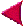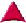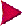Implementing AddressBookMore features# Writing iterators

In this section we are going to add our very own iterators to the class AddressBook. We will create two iterators, AddressBook#each and AddressBook#each_address, which can be used as usual:

 ``` address_book.each do |person| ... end address_book.each_address do |address| ... end ```

## Calling a block

When you write a function or method, you can call a code block with the yield keyword. Here is an example.

 ``` def twice yield yield end twice { puts "Hello World" } ```

This prints:

 ``` Hello World Hello World ```

## Passing parameters

You can use yield like any other method. To pass parameters to the code block, you simply pass them to yield. Take this example:

 ``` def names yield("Joe") yield("Sandy") yield("Melissa") end names do |name| puts "Hello " + name + ", how are you?" end ```

This prints:

 ``` Hello Joe, how are you? Hello Sandy, how are you? Hello Melissa, how are you? ```

You can pass as many parameters to the block as you like. For instance:

 ``` def full_names yield("Joe", "Smith") end full_names do |first,last| puts first + " " + last end ```

Prints:

 ``` Joe Smith ```

Now we can write our first iterator. AddressBook#each is the simplest of the two iterators. We step through every person in the array @persons and call yield on each one.

 ``` class AddressBook def each @persons.each { |p| yield p } end end ```

That's it. Now your class has an iterator.

 ``` class AddressBook def each_address @persons.each { |p| yield p.address } end end ```
 ``` class AddressBook # # Fundamental methods: initialize, add, remove # def initialize @persons = [] end def add(person) @persons += [person] @persons = @persons.sort{|a,b| by_name(a,b)} end def remove(person) @address.delete(person) end # # Iterators: each, each_address # def each @persons.each { |p| yield p } end def each_address @persons.each { |p| yield p.address } end # # Sorting function. # def by_name(a,b) if a.first_name == b.first_name a.last_name <=> b.last_name else a.first_name <=> b.first_name end end end ```Implementing AddressBookMore features